# Un peu de math

Using the latest Axelrod release to (fail to) reproduce Axelrod's tournament but investigating the replicator dynamics to get similar results

## Not reproducing Axelrod's first tournament

### 2019-05-24

In :
import axelrod as axl
import matplotlib.pyplot as plt
import numpy as np
import pandas as pd
%matplotlib inline


In 1980 Robert Axelrod ran two consecutive computer tournaments inviting people to submit computer code to play a Prisoners Dilemma tournament. He published the results of the first tournament in "Effective choice in the Prisoner’s Dilemma" but sadly none of the original computer code has survived.

Using the descriptions of the strategies given in the paper all but one of the strategies had been implemented in the Axelrod library but in the latest release (v4.6.0) of the Axelrod library the final remaining strategy (Graaskamp) has been implemented. In this post I'll show the results of reproducing (or at least attempting to reproduce) this first tournament.

First let us check we're running a sufficiently up to date version of the Axelrod library:

In :
from packaging import version
assert version.parse(axl.__version__) >= version.parse("4.6.0")
axl.__version__

Out:
'4.6.0'

In :
axl.seed(0)
first_tournament_participants_ordered_by_reported_rank = [
axl.TitForTat(),
axl.TidemanAndChieruzzi(),
axl.Nydegger(),
axl.Grofman(),
axl.Shubik(),
axl.SteinAndRapoport(),
axl.Grudger(),
axl.Davis(),
axl.RevisedDowning(revised=False),
axl.Feld(),
axl.Joss(),
axl.Tullock(),
axl.UnnamedStrategy(),
axl.Random()
]
number_of_strategies = len(first_tournament_participants_ordered_by_reported_rank)


Now let us create and run the tournament, repeating 500 times to smooth stochastic effects and using all available cores to copmpute things in parallel:

In :
number_of_turns = 200
number_of_repetitions = 500
tournament = axl.Tournament(
players=first_tournament_participants_ordered_by_reported_rank,
turns=number_of_turns,
repetitions=number_of_repetitions,
)
results = tournament.play(processes=0)

Playing matches: 100%|██████████| 120/120 [04:11<00:00,  1.23it/s]
Analysing: 100%|██████████| 25/25 [00:02<00:00,  9.38it/s]

In :
df = pd.DataFrame(results.summarise())

Out:
Rank Name Median_score Cooperation_rating Wins Initial_C_rate CC_rate CD_rate DC_rate DD_rate CC_to_C_rate CD_to_C_rate DC_to_C_rate DD_to_C_rate
0 0 Grofman 2.526429 0.847391 1.0 1.0 0.700922 0.146469 0.067779 0.084831 1.000000 0.286113 0.395632 0.987817
1 1 Shubik 2.476964 0.591424 4.0 1.0 0.570808 0.020616 0.088889 0.319687 1.000000 0.000000 0.444638 0.000000
2 2 Stein and Rapoport: 0.05: (D, D) 2.469643 0.587740 10.0 1.0 0.544663 0.043077 0.105794 0.306466 0.990018 0.217552 0.714150 0.000000
3 3 Nydegger 2.462857 0.995739 0.0 1.0 0.817494 0.178245 0.001741 0.002521 0.999742 0.990111 0.662257 0.642412
4 4 Tideman and Chieruzzi 2.458393 0.602221 4.0 1.0 0.585446 0.016775 0.076224 0.321554 1.000000 0.033630 0.403909 0.000000

## Has the results of Axelrod's first tournament been reproduced?¶

Here is the ranks of the strategies:

In :
results.ranked_names

Out:
['Grofman',
'Shubik',
'Stein and Rapoport: 0.05: (D, D)',
'Nydegger',
'Tideman and Chieruzzi',
'Grudger',
'Davis: 10',
'Tit For Tat',
'Revised Downing: False',
'Tullock: 11',
'Feld: 1.0, 0.5, 200',
'Unnamed Strategy',
'Joss: 0.9',
'Random: 0.5']

Here is a graphical look at this:

In :
plt.figure(figsize=(15, 6))

plt.plot((0, 15), (0, 15), color="grey", linestyle="--")

for original_rank, strategy in enumerate(first_tournament_participants_ordered_by_reported_rank):
rank = results.ranked_names.index(str(strategy))

if rank == original_rank:
symbol = "+"
plt.plot((rank, rank), (rank, 0), color="grey")
else:
symbol = "o"
plt.scatter([rank], [original_rank], marker=symbol, color="black", s=50)

plt.xticks(
range(number_of_strategies),
results.ranked_names,
rotation=90
)

plt.ylabel("Reported rank")
plt.xlabel("Reproduced rank");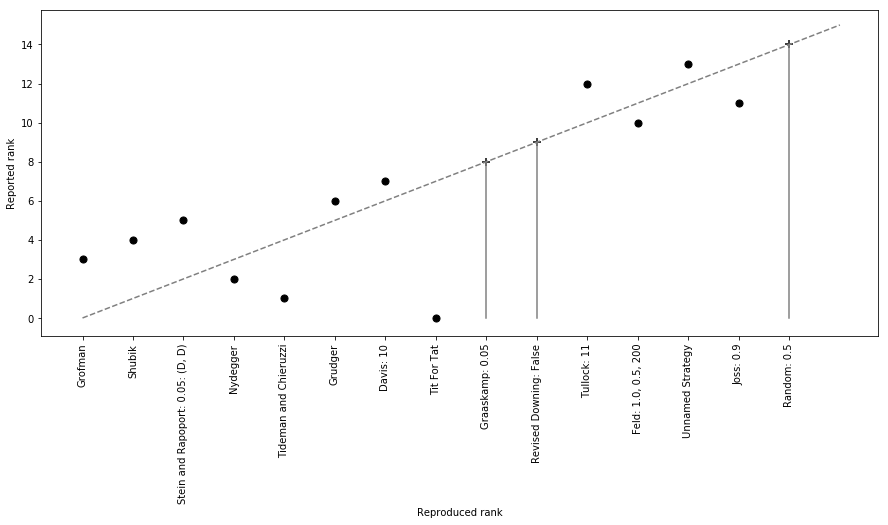We see that only 3 strategies are at the same rank as before:

Importantly, the very popular result stating that Tit For Tat won the tournament is not reproduced.

Below is a plot of the distribution of the average scores (per turn, per tournament) of each strategy:

In :
plot = axl.Plot(results)
plot.boxplot();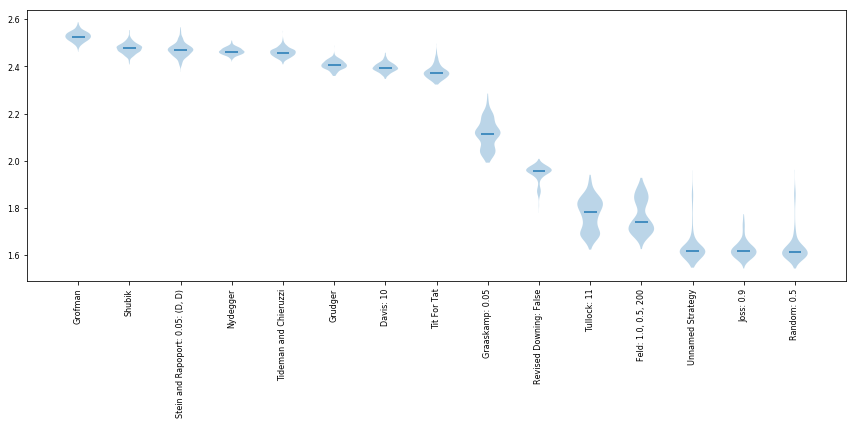We see that the first 8 strategy all perform very similarly but Tit For Tat does in fact rank 8th.

## Why do we not obtain the same results?¶

There are numerous reasons for which the results do not match Axelrod's original results:

• The strategies implemented do not correspond to the strategies used by Axelrod. As there is no source code available for the first tournament this is certainly a possibiliy. The strategies are not precisely described in the paper including some things like referring to a strategy that is not rigorously described: https://axelrod.readthedocs.io/en/stable/reference/all_strategies.html#axelrod.strategies.axelrod_first.Graaskamp
• The results reported by Axelrod are incorrect and/or where not repetead sufficiently to smooth out stochastic effects.
• Implementation errors: this is unlikely as the strategies are all open source, documented and automatically tested. Furthermore they were peer reviewed so multiple developers have looked over and confirmed their source code. Finally, if this is the reason for the results not matching: this would be good news. The library is a prime example of open science. If anyone reading this cares to look through the source code of the implemented strategies and point out an error: they can.

## Does this matter?¶

In some sense it's a slight pity that the results do not agree but an interesting question is whether or not this matters in terms of the conclusions made from Axelrod's paper.

One of the most important claims of the paper was that to do well a strategy needs to be "simple" (this is a slight simplification in itself). The description of the new winner is:

Cooperate on the first two rounds and returns the opponent's last action for the next 5. For the rest of the game Grofman cooperates if both players selected the same action in the previous round, and otherwise cooperates randomly with probability 2/7.

whilst this is not as simple a strategy as Tit For Tat it is still a strategy that fundamentally wants to cooperate.

## How does this impact the "emergence of cooperation"¶

Since Axelrod's work, one of the fundamental equations used to model the evolution of behaviour is the replicator dynamic equation:

$$\frac{dx_i}{dt} = x_i((Ax)_i - x^TAx) \text{ for all }i$$

where:

• $x\in\mathbb{R}^{n}$ and $0 \leq x_i \leq 1$ represents the proportion of strategy $i$ in the population for all $1 \leq i \leq n$
• $A\in\mathbb{R}^{n^2}$ is the utility matrix so that $A_{ij}$ represents the score/utility of strategy $i$ when interacting with strategy $j$.

We can solve this differential equation numerically:

In :
A = np.array(results.payoff_matrix)

import scipy.integrate

t = np.linspace(0, 10, 10_000)  # Obtain 10,000 time points

def dx(x, t=None, A=A):
"""
Define the derivate of x according
to the replicator dynamic equations
"""
return x * (A @ x - x @ A @ x.T)

xs = scipy.integrate.odeint(func=dx, y0=[1 / number_of_strategies for _ in A], t=t)

plt.figure(figsize=(15, 6))

plt.plot(xs)
plt.ylabel("$x_s$")
plt.xlabel("Time step");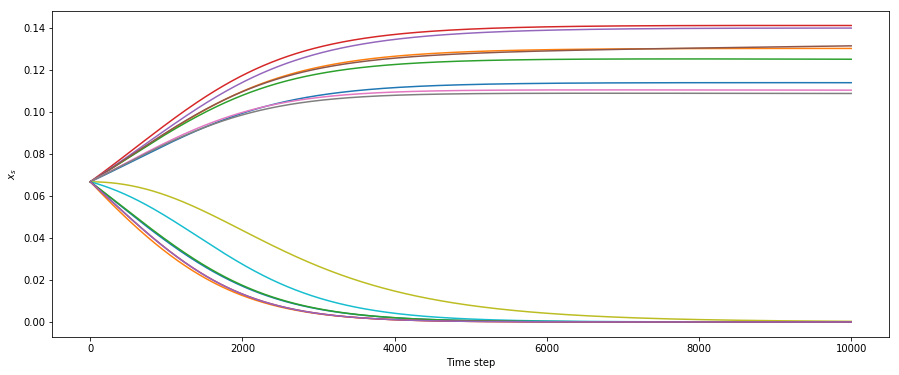Below is a plot of the probabilities of the last population:

In :
plt.figure(figsize=(15, 6))

plt.scatter(results.ranking, xs[-1],     marker="+",
color="black")
plt.xticks(
range(number_of_strategies),
results.ranked_names,
rotation=90
)
plt.ylabel("Stationary $x_i$")
plt.xlabel("Reproduced rank");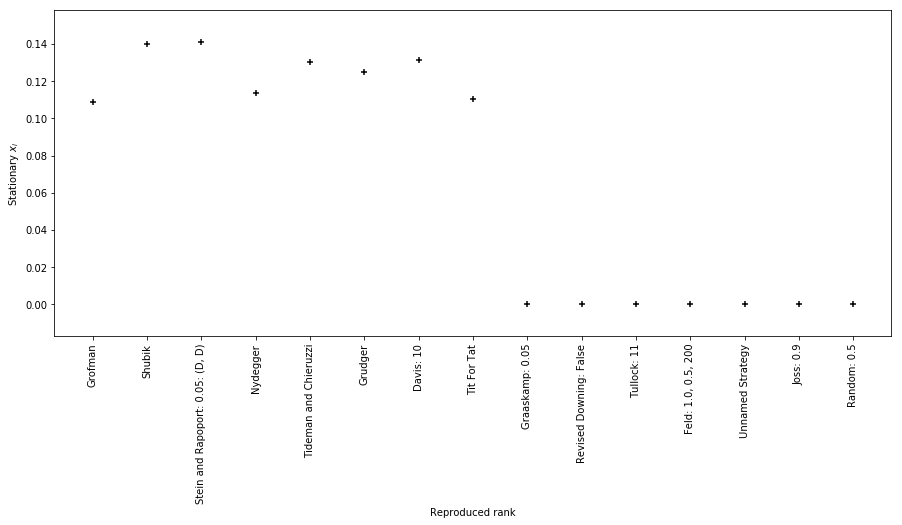We see that the top 8 ranking strategies (including Tit For Tat) all survive the evolutionary process.

## Does cooperation still emerge?¶

If we consider $C\in\mathbb{R}^{n^2}$ where $0 \leq C_{ij} \leq 1$ denotes the cooperation rate of strategy $i$ against strategy $j$, then the average cooperation rate in the population is given by:

$$x^T C x$$

Let us compute this value for all time steps in the numerical simulation and see how cooperation changes over time:

In :
C = np.array(results.cooperation) / (number_of_turns * number_of_repetitions)

plt.figure(figsize=(15, 6))
plt.plot([x @ C @ x.T for x in xs], color="black")
plt.ylabel("Cooperation rate")
plt.xlabel("Time step");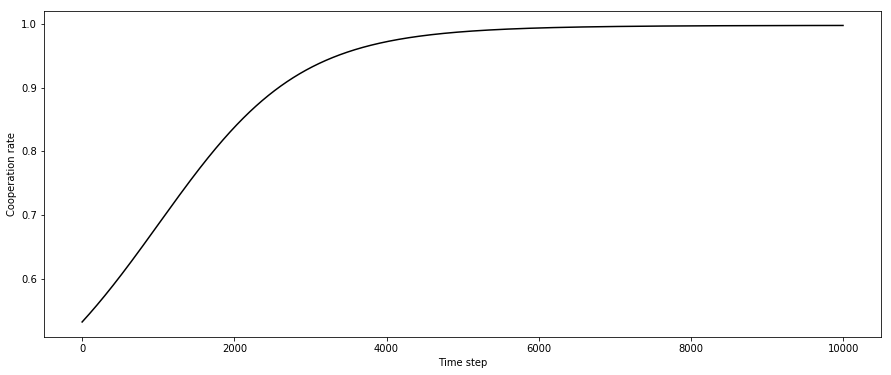As expected, over time, the cooperation rate tends to $1$ which implies that all the 8 top ranking strategies all essentially end up just cooperating with each other which confirms the emergence of cooperation.

Note

The source code from Axelrod's second tournament is available and we have revived that here: https://github.com/Axelrod-Python/axelrod-fortran. It is worth noting that apart from some minor discrepancies the results of that tournament do reproduce (and Tit For Tat wins). Owen Campbell (one of the core developers of the Axelrod library) gave a talk about this at PyCon UK in 2017: https://www.youtube.com/watch?v=OSFCvv2wpOU

A blog about programming (usually scientific python), mathematics (usually game theory) and learning (usually student centred pedagogic approaches).